# 【Python】Step Into Python Class

## Before All

Python作为一门`面向过程`兼容`面向对象`的语言，在面向对象中，使用`class`关键字来申明一个类。

But，是不是应该深入考虑一下这个`class`的底层实现过程呢？（不考虑CPython，仅仅考虑Python这一层）

## 申明一个类

``````class X:
pass
``````

``````class X:
def __init__(self, arg1, arg2) -> None:
self.arg1 = arg1
self.arg2 = arg2
``````

### 经典类与新式类

• 继承自`object`的，都是新式类

• 不继承`object`的，叫作经典类

• 因为Python3中，申明一个class的时候，默认继承了`object`

• 在Python2中，显式申明`class X(object)`，那么这个类就是新式类
• 如果没有显式申明，而是单纯的申明一个`class X:pass`，那么这个类就是经典类

`Python 2.1`之前，经典类是唯一可用的形式，在`Python 2.2`才引入了新式类。

## 继承

### 单继承

Python中想声明一个class继承了某个class，可以使用如下的方式：

``````class X(object):
pass
``````

### 多继承`多继承`有好处也有坏处，好处是可以灵活运用，坏处就是如果随意使用，很难看懂代码而且会存在一些属性冲突。

### Mixins

Mixins是一种规范，上述`socketserver`中的两个类就是使用了`Mixins`的规范：

• 需要混入使用的特性类，写在前面，例如这个`ThreadingMixIn`，就是需要混入使用的`线程类`
• 需要继承的父类放在最后，例如这个`UDPServer`，就是`ThreadingUDPServer`需要继承的父类
• 所有需要插入的Mixin特性的类以`MixIn`结尾

Minins只是一种开发规范，而不是硬性规定~

## 菱形继承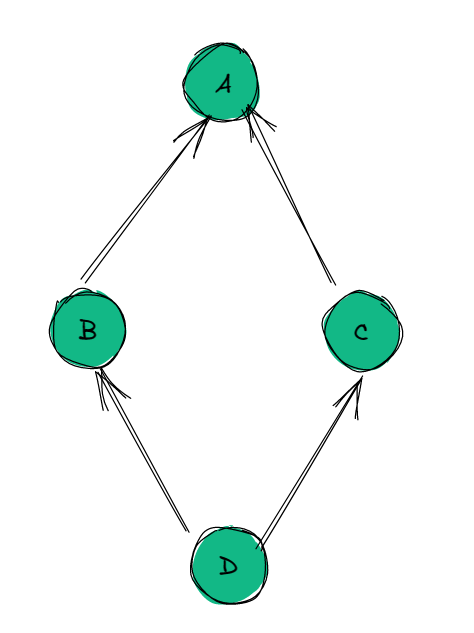``````class A:
pass

class B(A):
pass

class C(A):
pass

class D(B, C):
pass
``````

``````print(D.mro())
print(D.__mro__)
'''
[<class '__main__.D'>, <class '__main__.B'>, <class '__main__.C'>, <class '__main__.A'>, <class 'object'>]
(<class '__main__.D'>, <class '__main__.B'>, <class '__main__.C'>, <class '__main__.A'>, <class 'object'>)
'''
``````

• 经典类使用DFS
• Python 2.2 的新式类使用MRO
• Python 2.3 的新式类使用C3算法，同时也是Python3唯一支持的算法

### 经典类的DFS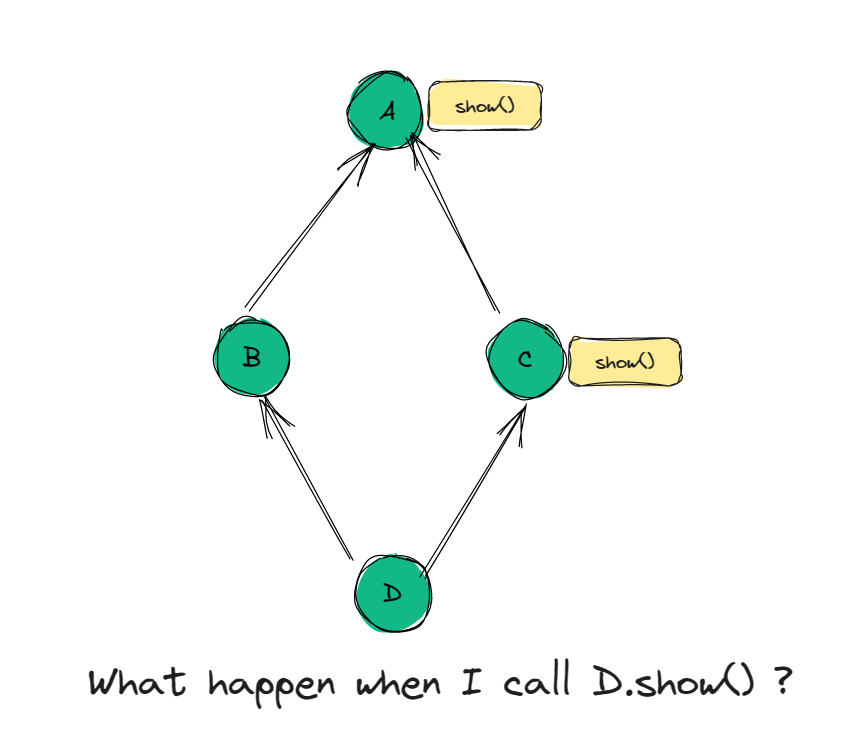• 由于是从左往右，先去找`B`有没有`show`，发现B没有，就去找`A`
• `A`直接找到了`show()`
• 所以`D`的对象会调用`A`中的`show()`

### 新式类的BFS

Python2.2后，引入了`新式类`，针对新式类，有一种全新的MRO方法

• `经典类`仍然使用`从左向右的DFS`
• `新式类`使用`从左想右的BFS`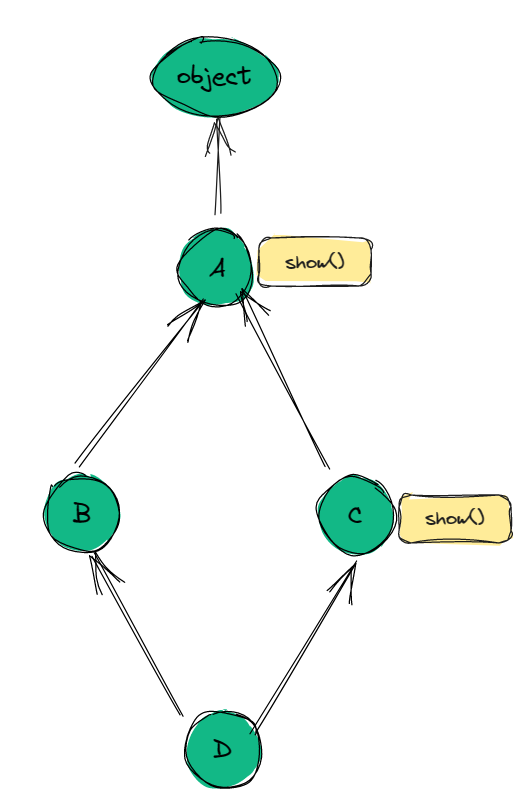`从左向右的BFS`算法的MRO顺序就是`[D,B,C,A,object]`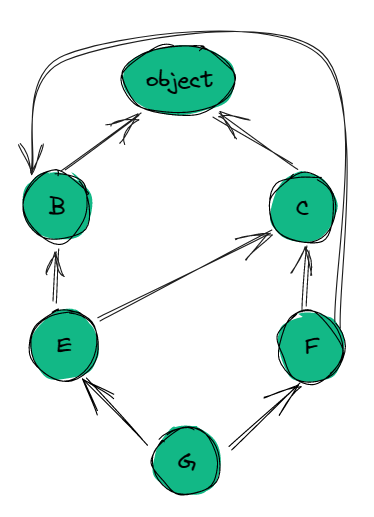``````class B:
pass

class C:
pass

class E(B, C):
pass

class F(C, B):
pass

class G(E, F):
pass
``````
• BFS(广度优先)的结果是`[G, E, F, B, C, object]`
• 对于`F`，搜索顺序是`[F, C , B, ojbect]` (object最后查找的原则)
• 对于`G`，搜索顺序是`[G, E, F, B, C, object]`
• F中的查询顺序是`CB` ，而G中的查询顺序是`BC`
• 上述的查询顺序违背了`单调性原则`，这个原则的意思是:
• 如果一个类X从父类X1和X2中派生出来，在MRO中，如果X1早于X2，那么在X的任何子类中都应该保持这个次序。
• 上述的F的子类G明显违背了这个原则！

### 新式C3算法

`Python 2.3`之后采用了`C3算法`来处理MRO

C3算法解决了`单调性问题``只能继承无法重写`问题，是基于`拓扑排序`的思想来解决问题的。

Python官方文档在2.3版本给出了相关的详细讲解The Python 2.3 Method Resolution Order | Python.org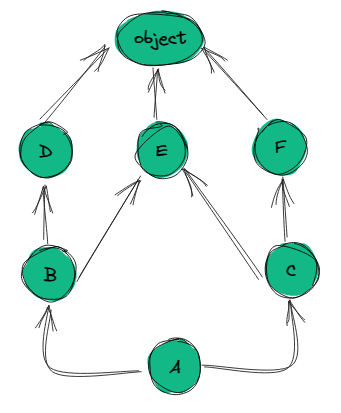• 造成这个的本直原因是——先查询了子类的父类，而另一个子类的方法重写没有生效，也就是DFS中存在的问题
• 把继承关系链看作一张`有向无环图`，使用`拓扑排序`的方式，构建一个`全序序列`，保证子类一定优先于父类被搜索

• 首先找图中`入度 == 0` 的点，刚开始只有`A`，选择`A`
• `A`的边删除，再找`入度 == 0` 的点，只有`B、C`
• 按照`最左原则`，选择`B`
• `B`的边删除，再找`入度 == 0` 的点，只有`D、C`
• 按照`最左原则`，选择`D`
• `D`的边删除，再找`入度 == 0` 的点，只有`C`
• 选择`C`，删除`C`的边
• 此时`入度 == 0`的点有`E、F`
• 按照`最左原则`，选择`E`
• `E`的边删除，再找`入度 == 0` 的点，只有`F`
• 选择`F`
• 最后选择`object`

C3算法就是在拓扑排序的基础上构建的，会对图中的每一个节点进行排序计算，一旦发现了逆序的存在就会产生一个`TypeError`

Graph ErrorTraceback (most recent call last):
File “e:/myworks/vscode_workspace/python_workspace/python_learning/class_learning.py”, line 13, in
class G(E, F):
TypeError: Cannot create a consistent method resolution
order (MRO) for bases B, C

• 检查第一个序列的头元素，记作 H。

• 若 H 未出现在其它列表的尾部，则将其输出，并将其从所有列表中删除，然后回到步骤1；否则，取出下一个列表的头部记作 H，继续该步骤。(这个步骤，相当于拓扑排序中的查找并删除入度为0的节点。)

• 重复上述步骤，直至列表为空或者 不能再找出可以输出的元素。如果是前一种情况，则算法结束；如果是后一种情况，说明无法构建继承关系(存在二义性继承)，Python 会抛出异常。

Graph C3 Codemro(D) = [D,O]
mro(E) = [E,O]
mro(F) = [F,O]
mro(B) = [B] + merge(mro(D),mro(E),[D,E])
= [B] + merge([D,O],[E,O],[D,E]) # E符合merge条件
= [B,D] + merge([O],[E,O],[D]) # D符合merge条件
= [B,D,E] + merge([O],[O],[]) # O符合merge条件
= [B,D,E,O]
= [C] + merge([E,O],[F,O],[E,F]) # E符合merge条件
= [C,E] + merge([O],[F,O],[F]) # F符合merge条件
= [C,E,F] + merge([O],[O],[]) # O符合merge条件
= [C,E,F,O]
= [A] + merge([B,D,E,O] ,[C,E,F,O] ,[B,C]) # B符合merge条件
= [A,B] + merge([D,E,O] ,[C,E,F,O] ,[C]) # D符合merge条件
= [A,B,D] + merge([E,O] ,[C,E,F,O] ,[C]) # C符合merge条件
= [A,B,D,C] + merge([E,O] ,[E,F,O] ,[]) # E符合merge条件
= [A,B,D,C,E] + merge([O] ,[F,O] ,[]) # F符合merge条件
= [A,B,D,C,E,F] + merge([O] ,[O] ,[]) # O符合merge条件
= [A,B,D,C,E,F,O]mro(B) = [B, O]
mro(E) = [E] + merge(mro(B), mro©, [B, C])
= [E] + merge([B, O], [C, O], [B, C])
= [E, B] + merge([O], [C, O], [C])
= [E, B, C] + merge([O], [O], [])
= [E, B, C, O]
mro(F) = [F] + merge(mro©, mro(B), [C, B])
= [F] + merge([C, O], [B, O], [C, B])
= [F, C] + merge([O], [B, O], [B])
= [F, C, B] + merge([O], [O], [])
= [F, C, B, O]
mro(G) = [G] + merge(mro(E), mro(F), [E, F])
= [G] + merge([E, B, C, O], [F, C, B, O], [E, F])
= [G, E] + merge([B, C, O], [F, C, B, O], [F])
= [G, E, F] + merge([B, C, O], [C, B, O], []) # 矛盾出现

## super的细节

super只能在`新式类`中使用！

``````class A:
def f1(self):
print("A f1")
super().f1()

class B:
def f1(self):
print("B f1")

class C(A, B):
pass

C().f1()
``````

• A f1

• B f1

C的mro是，`[<class '__main__.C'>, <class '__main__.A'>, <class '__main__.B'>, <class 'object'>]`

## Duck Typing

duck typing就是我们常说的`鸭子类型`

``````from collections.abc import Iterable

class MyIter:
def __iter__(self):
pass

print(issubclass(MyIter, Iterable))	# True
``````

``````class Iterable(metaclass=ABCMeta):

__slots__ = ()

@abstractmethod
def __iter__(self):
while False:
yield None

@classmethod
def __subclasshook__(cls, C):
if cls is Iterable:
return _check_methods(C, "__iter__")
return NotImplemented
``````
• Iterable定义自己的`元类``ABCMeta`，有关元类的知识点之后再讨论。
• Iterable定义了一个`abstractmethod`，这个抽象方法装饰器的作用就是，如果一个类显式继承`Iterable`，那么这个类一定要有一个`__iter__`的方法，否则会报错
• Iterable定义了一个`classmethod`叫作`__subclasshook__`，这个方法在调用`issubclass()`函数的时候会被调用，检测C这个是否含有`__iter__`这方法，如果有，就返回True，那么在调用`issubclass``isinstance`的时候就会返回True，证明是子类

``````from abc import ABC

class MyBaseClass(ABC):
@classmethod
def __subclasshook__(cls, subclass):
print(cls)
print(subclass)
return hasattr(subclass, 'my_method') and callable(subclass.my_method)

class MySubClass:
def my_method(self):
print("Hello, World!")

print(issubclass(MySubClass, MyBaseClass)) 	# True
print(isinstance(MySubClass(), MyBaseClass))# True
``````

## 抽象基类

``````from abc import ABC, abstractmethod

class MyBaseClass(ABC):
@abstractmethod
def need_this_func(self):
pass

@classmethod
def __subclasshook__(cls, subclass):
print(cls)
print(subclass)
return hasattr(subclass, 'my_method') and callable(subclass.my_method)

class MySubClass(MyBaseClass):
def my_method(self):
print("Hello, World!")

MySubClass()
'''
Traceback (most recent call last):
File "e:/myworks/vscode_workspace/python_workspace/python_learning/class_learning.py", line 88, in <module>
MySubClass()
TypeError: Can't instantiate abstract class MySubClass with abstract methods need_this_func
'''
``````

## 类方法与静态方法

``````class X:
@classmethod
def instance(cls):
if hasattr(cls, "_obj"):
return cls._obj
cls._obj = X()
return cls._obj

print(X.instance())
print(dir(X))
'''
<__main__.X object at 0x000001EB271CFD30>
['__class__', '__delattr__', '__dict__', '__dir__', '__doc__', '__eq__', '__format__', '__ge__', '__getattribute__', '__gt__', '__hash__', '__init__', '__init_subclass__', '__le__', '__lt__', '__module__', '__ne__', '__new__', '__reduce__', '__reduce_ex__', '__repr__', '__setattr__', '__sizeof__', '__str__', '__subclasshook__', '__weakref__', '_obj', 'instance']
'''
``````

``````class Util:
@staticmethod
def date():
return "20230531"

print(Util.date())	# "20230531"
``````

## metaclass

`metaclass`翻译成中文，就是`元类`

### type元类

``````print(type(str(1)))
print(type(str))
print(type(int("1")))
print(type(int))
print(type(list("123")))
print(type(list))
print(type(tuple("123")))
print(type(tuple))
'''
<class 'str'>
<class 'type'>
<class 'int'>
<class 'type'>
<class 'list'>
<class 'type'>
<class 'tuple'>
<class 'type'>
'''
``````

• 所有由基本类型构建的对象执行`type()`后，都指向这个类
• 基本类型的`type()`执行后，返回的都是`<class 'type'>`
• 这个`<class 'type'>`其实就是`metaclass`，也就是`元类`

• 元类：`实例化产生类的类`
• 元类 --> 元类.实例化() --> 类 --> 类.实例化() --> 对象

``````class_name = "Wood"     # 类名
class_bases = (object, )# 基类
class_dic = {}
class_body = '''
def __init__(self):
pass
def info(self):
print("test info")
'''
exec(class_body, {}, class_dic) # 使用exec将class_body中的内容装入class_dic中
Wood = type(class_name, class_bases, class_dic)
obj = Wood()
obj.info()
print(dir(obj))
print(type(obj))

'''
test info
['__class__', '__delattr__', '__dict__', '__dir__', '__doc__', '__eq__', '__format__', '__ge__', '__getattribute__', '__gt__', '__hash__', '__init__', '__init_subclass__', '__le__', '__lt__', '__module__', '__ne__', '__new__', '__reduce__', '__reduce_ex__', '__repr__', '__setattr__', '__sizeof__', '__str__', '__subclasshook__', '__weakref__', 'info']
<class '__main__.Wood'>
'''
``````

• class关键字到底做了什么来构建了一个class？
• 元类充当了什么样的一个角色？

• 获取类名
• 获取基类（父类）
• 获取名称空间
• 调用元类构建这个类

• 元类的实例化对象是一个`类`

### 自定义元类

``````class MyMetaclass(type):
def __init__(self, *args, **kwargs):
print(args)
print(kwargs)
print(self)
print(self.mro())
print(dir(self))

class X(metaclass=MyMetaclass):
def func1(self):
pass

X()
'''
('X', (), {'__module__': '__main__', '__qualname__': 'X', 'func1': <function X.func1 at 0x000001D969F9C1F0>})
{}
<class '__main__.X'>
[<class '__main__.X'>, <class 'object'>]
['__class__', '__delattr__', '__dict__', '__dir__', '__doc__', '__eq__', '__format__', '__ge__', '__getattribute__', '__gt__', '__hash__', '__init__', '__init_subclass__', '__le__', '__lt__', '__module__', '__ne__', '__new__', '__reduce__', '__reduce_ex__', '__repr__', '__setattr__', '__sizeof__', '__str__', '__subclasshook__', '__weakref__', 'func1']
'''
``````

1. 类名
2. 基类（父类）
3. 命名空间

• 如果仅仅是在`__init__`中注入一些属性，那么如何解释传入的参数中不存在`object`，但是`self.mro()`中又有`object`了？
• Python3中都是新生类，如何保证class申明后不显式继承`object`也会自动继承`object`
• 有什么方法是在`__init__`之前就执行的？

### __new__

``````class MyMetaclass(type):
def __init__(self, *args, **kwargs):
print("-"*50)
print(args)
print(kwargs)
print(self)
print(self.mro())
print(dir(self))

def __new__(cls, *args, **kwargs):
print(cls)
print(args)
print(kwargs)
return super().__new__(cls, *args, **kwargs)

class X(metaclass=MyMetaclass):
def func1(self):
pass

x = X()
``````

``````<class '__main__.MyMetaclass'>
('X', (), {'__module__': '__main__', '__qualname__': 'X', 'func1': <function X.func1 at 0x0000013C3A89C280>})
{}
--------------------------------------------------
('X', (), {'__module__': '__main__', '__qualname__': 'X', 'func1': <function X.func1 at 0x0000013C3A89C280>})
{}
<class '__main__.X'>
[<class '__main__.X'>, <class 'object'>]
['__class__', '__delattr__', '__dict__', '__dir__', '__doc__', '__eq__', '__format__', '__ge__', '__getattribute__', '__gt__', '__hash__', '__init__', '__init_subclass__', '__le__', '__lt__', '__module__', '__ne__', '__new__', '__reduce__', '__reduce_ex__', '__repr__', '__setattr__', '__sizeof__', '__str__', '__subclasshook__', '__weakref__', 'func1']
``````

• 每个`class`，都是由`__new__`构造出来的
• `__new__`早于`__init__`

• 有一个魔术方法叫作`__call__`，就是一个`类的对象`在被调用的时候会执行的

### __call__

``````class TestClass:
def __init__(self, name) -> None:
self.name = name

def __call__(self, *args, **kwds):
print(self.name)

obj = TestClass("woodwhale")
obj()	# woodwhale
``````

• `__call__`函数是可以有返回值的，当作一个函数就好理解了
• `__call__`函数还可以有参数，这点也和函数一样

``````class_name = "TestClass"
class_bases = (object, )
class_dic = {}
class_body = '''
def __init__(self):
pass
def info(self):
print("test info")
'''
exec(class_body, {}, class_dic)
TestClass = type(class_name, class_bases, class_dic)
``````
• 既然`type`是一个类，那么在调用`type()`的时候，其实触发的就是`type`这个类的`__call__`方法
• 也就是说，在执行`type(class_name, class_bases, class_dic)`的时候，其实是在`__call__`函数中返回了一个`类对象`

• 其实就是因为最基本的类的元类是`type`，而在`type``__call__`函数中，会调用这个最基本类的`__new__``__init__`

``````class MyMetaclass(type):

def __call__(self, *args, **kwds):
print(self)
print("__call__")
return "woodwhale"

class X(metaclass=MyMetaclass):
def func1(self):
pass
print(X())
'''
<class '__main__.X'>
__call__
woodwhale
'''
``````

1. `X()`的第一步会去先实例化一个`MyMetaclass`
2. `MyMetaclass`的实例化对象是`X`
3. `X()`其实就是`MyMetaclass`实例化对象被调用了，所以会触发`MyMetaclass``__call__`函数
4. `MyMetaclass``__call__`中，返回值是`woodwhale`
5. 所以最后`print(X())`的结果是`woodwhale`

``````class MyMetaclass(type):

def __call__(self, *args, **kwargs):
print(self)
print("__call__")
x_obj = self.__new__(self)
self.__init__(self, *args, **kwargs)
return x_obj

class X(metaclass=MyMetaclass):

def __new__(cls, *args, **kwargs):
# return object.__new__(cls)
obj = super().__new__(cls)
# 随意操作！
return obj

def __init__(self, name) -> None:
print("__init__")
self.name = name

def func1(self):
pass
x = X("123")
print(x.name)
``````

## 属性查找

• 对象 --> 类 --> 父类 --> object
• 找不到就报错

1. 对于对象
2. 对于类

• 对象 --> 类 --> 父类 --> object
• 找不到就报错

• 对象 --> 类 --> 父类 --> object --> 自定义的metaclass --> type
• 找不到就报错